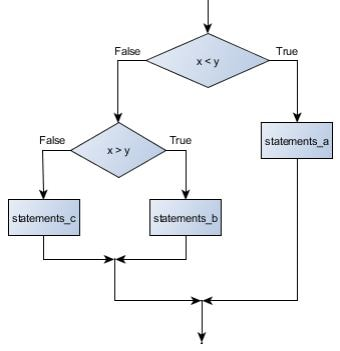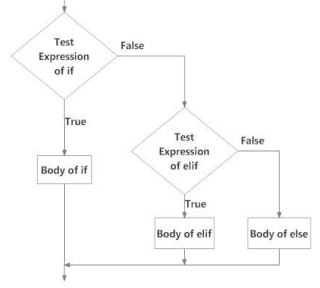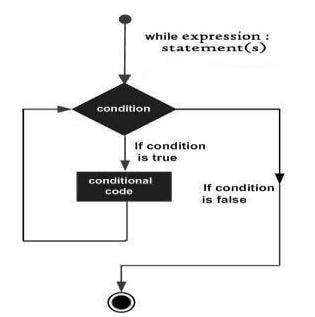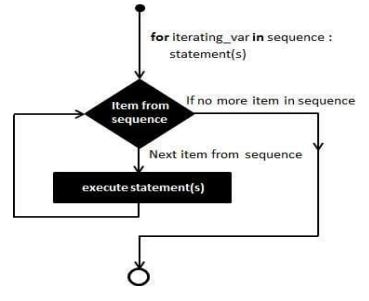Special Offer - Enroll Now and Get 2 Course at ₹25000/- Only Explore Now!# Python Control Flow

May 20th, 2019

## Flow Control

Control flow (or alternatively, flow of control) refers to the specification of the order in which the individual statements, instructions or function calls of a program are executed or evaluated. In order to control the flow of a program, we have conditional programming and looping.

### Conditional Programming

Python provides conditional branching with if statements and looping with while and for statements. Python also has a conditional expression—this is a kind of if statement that is Python’s answer to the ternary operator (?:) used in C-style languages. Please find below example for the conditional flow of statements.

#### Flowchart for conditional execution of statementsIn the above flow chart, it shows a control flow of statements based upon the given inputs of x and y
values.

### Conditional Operators

• > – Greater
• < – Lesser
• == – equal
• <= – Lesser or equal
• >= – Greater or equal
• != – Not equal

• True = 1
• False = 0

### Logical Operators

• and – logical AND operation
• or – logical OR operation

### If …elif…else Conditional Statements

It evaluates an expression and, based on the result, choose which part of the code to execute
(decision making is required when we want to execute code only if a certain condition is satisfied).

 ```if boolean_expression1 Statement1 elif boolean_expression2: Statement2 . . else: Default Statement```
There can be zero or more elif clauses and the final else clause is optional. In some cases, we
can reduce an if…else statement down to a single conditional expression.

##### The syntax for a conditional expression is:
`expression1 if boolean_expression else expression2`

In above example you can see that the program is dependent on the boolean_expression, If the
boolean_expression evaluates to True, the result of the conditional expression is expression1; otherwise, the
result is expression2.

##### Syntax of if…elif…else:
```if test expression:
Body of if
elif test expression:
Body of elif
else:
Body of else```

#### Flowchart of if…elif…elseIn below example we are going to validate a numerical value using if..elif..else.

##### Example 1: test1.py
 ```# Program checks if the number is positive or negative # And displays an appropriate message num = 3 if num == 0: print ("Zero") elif num > 0: print (“Positive number”) else: print ("Negative number")```
(Create a file and copy above script and execute using python. Change the value of “num” and verify different
combinations.)

### Ternary operator

The short version of an if/else. When the value of a name is to be assigned according to some condition, sometimes it’s easier and more readable to use the ternary operator instead of a proper if clause. In the following example, the two code blocks do exactly the same thing:

##### Example :
 ```ternary.py # python script order_total = 247 # classic if/else form if order_total > 100: discount = 25 else: discount = 0 print(order_total, discount) # ternary operator discount = 25 if order_total > 100 else 0 print(order_total, discount)```

### Repetition Structures

A repetition structure causes a statement or set of statements to execute repeatedly. Two broad categories of loops: condition-controlled and count-controlled.

• A condition-controlled loop uses a true/false condition to control the number of
times that it repeats.
• A count-controlled loop repeats a specific number of times.

In Python, you use the while statement to write a condition-controlled loop, and you use
the for the statement to write a count-controlled loop.

### Condition-Controlled Loop

The while loop gets its name from the way it works: while a condition is true, do some

#### The loop has two parts

• A condition that is tested for a true or false value
• A statement or set of statements that is repeated as long as the condition is true.

#### Flowchart for Condition Controlled LoopIn the above figure you can identify the logic of a while loop.

##### The while statement has the general form:(SYNTAX)
```while condition :
Code block
(Or) statements```
•  The reserved word “while” begins the while statement.
•  The “condition” determines whether the body will be (or will continue to be) executed.
• A colon (:) must follow the condition.
• Code bloc is a block of one or more statements to be executed as long as the condition is true.

As a block, all the statements that comprise the block must be indented the same number of spaces from the left. As with the if statement, the block must be indented more spaces than the line that begins the while statement. The block technically is part of the while statement. The below example will give the usage of while.

##### Example:
 ```natural_number_sum.py # Program to add natural # sum = 1+2+3+...+n n = 10 # initialize sum and counter sum = 0 i = 1 while i <= n: sum = sum + i i = i+1 # update counter # print the sum print("The sum is", sum)```

### Infinite Loops

An infinite loop continues to repeat until the program is interrupted. If a loop does not have a way of
stopping, it is called an infinite loop. Please find the below example:

##### Example: Infinity.py

# This program demonstrates an infinite loop.
# Create a variable to control the loop.
keep_going = ‘y’
# Warning! Infinite loop!
while keep_going == ‘y’:
# Get a salesperson’s sales and commission rate.
sales = float(input(‘Enter the amount of sales: ‘))
comm_rate = float(input(‘Enter the commission rate: ‘))
# Calculate the commission.
commission = sales * comm_rate
# Display the commission.
print(‘The commission is \$’.format(commission, ‘,.2f’), sep=’ ‘)

### Count-Controlled Loop

A count-controlled loop iterates a specific number of times. In Python, you use the for statement to
write a count-controlled loop. In Python, the for statement is designed to work with a sequence of data
items. When the statement executes, it iterates once for each item in the sequence.

##### Here is the general format: SYNTAX
```for variable in [value1, value2, etc.]:
statement
statement```

#### Flow chart of “for” loop:The for statement iterates over a range of values. These values can be a numeric range, or, as we shall, elements of a data structure like a string, list, or tuple.

##### Example: test_for.py
 ```for n in range(1, 11): print(n)```
Note: The expression range(1, 11) creates an object known as an iterable that allows the for loop to assign
to the variable n the values 1, 2, . . . , 10. During the first iteration of the loop, n’s value is 1 within
the block. In the loop’s second iteration, n has the value of 2.

##### Syntax:
`range( begin,end,step )`

where,

•  begin is the first value in the range; if omitted, the default value is 0
•  the end is one past the last value in the range; the end value may not be omitted
•  change is the amount to increment or decrement; if the change parameter is omitted, it defaults to 1
(counts up by ones).
• begin, end, and step must all be integer values; floating-point values and other types are not allowed.
##### Example: simple_loop.py
 ```# This program demonstrates a simple for loop # that uses a list of numbers. print('I will display the numbers 1 through 5.') for num in [1, 2, 3, 4, 5]: print(num)```